# Chapter 3: Principles of Power System¶

### Example 3.1, Page Number: 50¶

In :
#Variable declaration:
M = 100                          #Maximum demand on power station(MW)
L = 0.40                         #annual load factor

#Calculation:
E = M*L*8760*10**6              #Energy generated in a year(kWh)

#Results:
print "Energy generated in a year is (",E/10**8,"* 10^5) kWh"

Energy generated in a year is ( 3504.0 * 10^5) kWh


### Example 3.2, Page Number: 50¶

In :
from __future__ import division

#Variable declaration:
M = 20                      #Maximum demand(MW)
E = 61.5*10**6              #Units genearted per annum(kWh)

#calculation:
DF = M/L                    #demand factor

#Results:
print "The demand factor is",round(DF,3)

The demand factor is 0.465


### Example 3.3, Page Number: 50¶

In :
from __future__ import division

#variable declaration:

#power station delivers 100 MW for 2 hours,
#50 MW for 6 hours and is shut down for the rest
#of each day.
M = 100                       #Maximum capacity of station(MW)

#Calculation:
E1 = 100*2+50*6                #Energy supplied per day(MWh)
n = 365-45                     #No.of working days


Annual load factor is 20.8 %


### Example 3.4, Page Number: 50¶

In :
from __future__ import division

#Variable declaration:
M = 25                      #Maximum demand(MW)
PCF = 0.50                  #plant capacity factor
PUF = 0.72                  #plant use factor

#Calculations:
PC = L/PCF                  #Plant gapacity(MW)
R = PC-M                    #Reserve capacity(MW)
E = L*24                    #daily energy produced(MWh)
ME = E/PUF                  #Maximum energy produced(MWh/day)

#Results:
print "Reserve capacity is ",R,"MW"
print "Daily energy produced is",E,"MWh"
print "Maximum energy that could be produced dauly is ",ME,"MWh/day"

Reserve capacity is  5.0 MW
Daily energy produced is 360.0 MWh
Maximum energy that could be produced dauly is  500.0 MWh/day


### Example 3.5, Page Number: 51¶

In :
from __future__ import division

#Variable declaration:
M = 2500                 #Maximum demand(kW)
E = 45*10**5             #kWh/year

#Calculation:
D = (1500+750+100+450)/M    #diversity factor
LF = E/(M*8760)              #Annual load factor

#Results:
print "Diversity factor is",D

Diversity factor is 1.12
Annual load factor is 20.5 %


### Example 3.6, Page Number: 51¶

In :
from __future__ import division

#Variable declaration:
M = 15000              #Max demand(kW)
LF = 0.50              #Annual load factor
PCF = 0.4              #Plant capacity factor

#Calculations:
PC = L/PCF             #plant capacity(kW)
R = PC-M               #Reserve capacity(kW)

#Results:
print "The reserve capacity is ",R,"kW"

The reserve capacity is  3750.0 kW


### Example 3.7, Page Number: 51¶

In :
from __future__ import division

#Variable declaration:
DFo = 1.35              #Overall diversity factor of system

#Calculations:
M = (1500+2000+10000)/DFo    #max demand(kW)
CL1 = (1500*1.2)/0.8          #kW

CL2 = 2000*1.1/0.9             #kW

CL3 = 10000*1.25/1             #kW

#Results:
print "Maximum demand is ",M,"kW"
print "Connected loads for each type are:"
print CL1,"kW ",round(CL2),"kW ",CL3,"kW"

Maximum demand is  10000.0 kW
Connected loads for each type are:
2250.0 kW  2444.0 kW  12500.0 kW


### Example 3.8, Page Number: 52¶

In :
#Variable declaration:
Dt = 1.3             #Diversity among transformers

#Calculation:
#for transformer 1:
M1 = (10*0.65)/1.5            #Max demand (kW)

#for transformer 2:
M2 = (12*0.6)/3.5            #max demand(kW)

#for transformer:
M3 = (15*0.7)/1.5             #max demand(kW)

Mf = (M1+M2+M3)/Dt           #Maximum demand on feeder(kW)

#Results:
print "Maximum load on the feeder is ",round(Mf,1),"kW"

Maximum load on the feeder is  10.3 kW


### Example 3.9, Page Number: 52¶

In :
#Variable declaration:
n1 = 1000               #No of houses
CL1 = 1.5               #Avg connected load in each house(kW)
DF1 = 0.4                #Demand factor
DvF = 2.5               #Diversity factor

n2 = 10                  #no. of factories
M2 = 90                 #factory overall max demand(kW)

n3 = 7                  #no of tubewells
C3 = 7                  #capacity of tubewells(kW)

DF = 1.2                #Diversity factor among above types of consumers

#Calculations:
Ms = ((DF1*n1*CL1)/DvF)+M2+n3*C3      #Sum of maximum demand on the station(kW)
M = Ms/DF                             #maximum demand on the station(kW)

#Results:
print "Minimum capacity on the power station is",round(M),"kW"

Minimum capacity on the power station is 316.0 kW


### Example 3.10, Page Number: 53¶

In :
from __future__ import division
%matplotlib inline

#Calculations:
M = 70.0                      #Max demand, from load curve(MW)
E = 40*6+50*4+60*2+50*4+70*4+40*4        #Units generated per day(MWh)

n1 = linspace(0,6,10);
M1 = linspace(40,40,10);
plot(n1,M1);
hold(True);

n2 = linspace(6,10,10);
M2 = linspace(50,50,10);
plot(n2,M2,'b');

n3 = linspace(10,12,10);
M3 = linspace(60,60,10);
plot(n3,M3,'b');

n4 = linspace(12,16,10);
M4 = linspace(50,50,10);
plot(n4,M4,'b');

n5 = linspace(16,20,10);
M5 = linspace(70,70,10);
plot(n5,M5,'b');

n6 = linspace(20,24,10);
M6 = linspace(40,40,10);
plot(n6,M6,'b');

ylim(0,100);
xlim(0,24);
grid(1,linewidth=0.5);
xlabel("Time in hours   ----->");

#Results:
print "Maximum demand is ",M,"MW"
print "Units generated per day is (",E/100,"* 10^5)  kWh"
print "The Daily load curve is shown below:"

Populating the interactive namespace from numpy and matplotlib
Maximum demand is  70.0 MW
Units generated per day is ( 12.0 * 10^5)  kWh
The Daily load curve is shown below: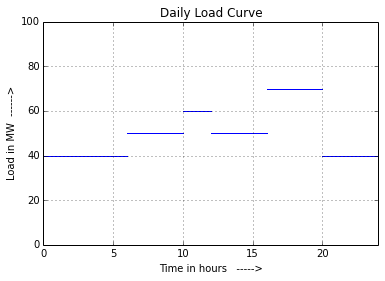### Example 3.11, Page Number: 54¶

In :
from __future__ import division
%matplotlib inline

#Calculation:
M = 350.0                             #Maximum demand(kW)
DF = (200+100+50+100)/M        #Diversity factor
E = 100*6+(100+50)*2+(200+100+50)*2+(200+100)*8+100*6    #kWh/day

n1 = linspace(0,6,10);
M1 = linspace(100,100,10);
plot(n1,M1);

hold(True);

n2 = linspace(6,8,10);
M2 = linspace(150,150,10);
plot(n2,M2,'b');

n3 = linspace(8,10,10);
M3 = linspace(350,350,10);
plot(n3,M3,'b');

n4 = linspace(10,18,10);
M4 = linspace(300,300,10);
plot(n4,M4,'b');

n5 = linspace(18,24,10);
M5 = linspace(100,100,10);
plot(n5,M5,'b');

ylim(0,400);
xlim(0,24);
grid(linewidth=0.5);
xlabel("Time in hours   ----->");

#Results:
print "Diversity factor is ",round(DF,3)
print "Unis generated per day is ",E,"kWh"
print "The daily load curve is shown below:"

Populating the interactive namespace from numpy and matplotlib
Diversity factor is  1.286
Unis generated per day is  4600 kWh
The daily load curve is shown below: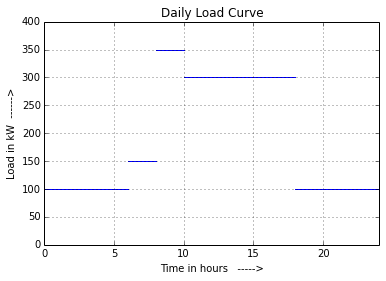### Example 3.12, Page Number: 54¶

In :
from __future__ import division
%matplotlib inline

#Calculation:
M1 = 800                     #max demand of consumer 1(Watts)
M2 = 1000                    #max demand of consumer 2(Watts)
M3 = 1200                    #max demand of consumer 3(Watts)

n1 = linspace(0,8,10);
m1 = linspace(200,200,10);
plot(n1,m1);

hold(True);
n2 = linspace(8,14,10);
m2 = linspace(800,800,10);
plot(n2,m2,'b');

n3 = linspace(14,16,10);
m3 = linspace(2400,2400,10);
plot(n3,m3,'b');

n4 = linspace(16,22,10);
m4 = linspace(800,800,10);
plot(n4,m4,'b');

n5 = linspace(22,24,10);
m5 = linspace(400,400,10);
plot(n5,m5,'b');

ylim(0,2500);
xlim(0,24);
grid(linewidth=0.5);
xlabel("Time in hours   ----->");
annotate("(Midnight)",xy=(0,0));
annotate("(Midnight)",xy=(24,0));
annotate("(Noon)",xy=(12,0));

LF1 = (600*6+200*2+800*6)/(24*M1)
LF2 = (200*8+1000*2+200*2)/(24*M2)
LF3 = (200*6+1200*2+200*2)/(24*M3)
#The simultaneous maximum demand on the station is 200 + 1000 + 1200 = 2400 W
#and occurs from 2 P.M. to 4 P.M.
DF = (M1+M2+M3)/2400.0           #Diversity factor

#Results:
print "(i)  The maximum demand of individual consumers are:"
print "\tConsumer 1 =",M1,"W, Consumer 2 =",M2,"W, Consumer 3 =",M3,"W"
print "\n(ii)Load Factors of individual consumers are:"
print "\tConsumer 1 =",round(LF1*100,1),"%, Consumer 2 =",round(LF2*100,1),"""%,
\tConsumer 3 =""",round(LF3*100,1),"%"
print "\n(iii)Diversity factor is ",DF
print "\n(iv) Load factor of the station is",round(LF*100,1),"%"
print "\n   The load curve is shown below:"

Populating the interactive namespace from numpy and matplotlib
(i)  The maximum demand of individual consumers are:
Consumer 1 = 800 W, Consumer 2 = 1000 W, Consumer 3 = 1200 W

(ii)Load Factors of individual consumers are:
Consumer 1 = 45.8 %, Consumer 2 = 16.7 %,
Consumer 3 = 13.9 %

(iii)Diversity factor is  1.25

(iv) Load factor of the station is 29.2 %

The load curve is shown below: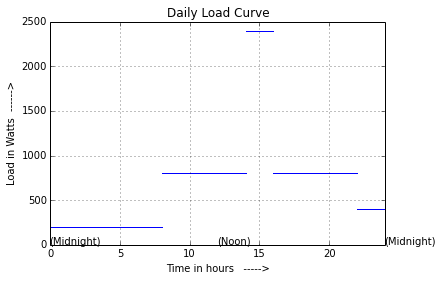### Example 3.13, Page Number: 55¶

In :
from __future__ import division

#Variable declaration:
A = 12                     #Area of load curve(cm**2)
Lo = 1000                  #load under 1cm length(kW)
To = 2                     #time under 1cm length(hr)
M = 3000                   #maximum demand(kW)

#Calculation:

#Results:

Load factor is 33.3 %


### Example 3.14, Page Number: 56¶

In :
from __future__ import division

#Variable declaration:
C = 75                      #Capacity of each generator(MW)
n = 4                       #No. of generators
CV = 10000                  #Calorific value of oil used(kcal/kg)
H = 2860                    #Avg heat rate(kcal/kWh)

#Calculation:
E = (260*6+200*8+160*4+100*6)            #Units generated per day(MWh)
PCF = L/(n*C)               #Plant capacity
TH = H*E*10**3              #heat generated per day(kcal)
w = TH/CV                   #daily fuel requirement(kg)

print "Plant capacity factor is",round(PCF*100,1),"%"
print "Daily fuel requirement is (",w/1000,"* 10^3)  kg"

Daily load factor is 70.5 %
Plant capacity factor is 61.1 %
Daily fuel requirement is ( 1258.4 * 10^3)  kg


### Example 3.15, Page Number: 56¶

In :
from __future__ import division
%matplotlib inline

#Calculations:
E = 20*2+40*4+60*4+20*4+50*4+20*6    #Units generated per day(MWh)

#1st plot
subplot(1,3,1)
n1 = linspace(0,8,10);
m1 = linspace(20,20,10);
plot(n1,m1);

hold(True);
n2 = linspace(8,12,10);
m2 = linspace(40,40,10);
plot(n2,m2,'b');

n3 = linspace(12,16,10);
m3 = linspace(60,60,10);
plot(n3,m3,'b');

n4 = linspace(16,20,10);
m4 = linspace(20,20,10);
plot(n4,m4,'b');

n5 = linspace(20,24,10);
m5 = linspace(40,40,10);
plot(n5,m5,'b');

ylim(0,100);
xlim(0,24);
grid(linewidth=0.5);
xlabel("Time in hours   ----->");
title("$(i)$ Daily Load Curve");

#next plot
subplot(1,3,3)
n1 = linspace(0,4,10);
m1 = linspace(60,60,10);
plot(n1,m1);

hold(True);
n2 = linspace(4,8,10);
m2 = linspace(50,50,10);
plot(n2,m2,'b');

n3 = linspace(8,12,10);
m3 = linspace(40,40,10);
plot(n3,m3,'b');

n4 = linspace(12,24,10);
m4 = linspace(20,20,10);
plot(n4,m4,'b');

ylim(0,100);
xlim(0,24);
grid(linewidth=0.5);
xlabel("Hours duration  ----->");
title("$(ii)$ Load Duration Curve");

#Results:
print "Energy generated per day is (",E,"* 10^3)  kWh"
print "\nThe daily load curve and load duration curves are shown below:"

Populating the interactive namespace from numpy and matplotlib
Energy generated per day is ( 840 * 10^3)  kWh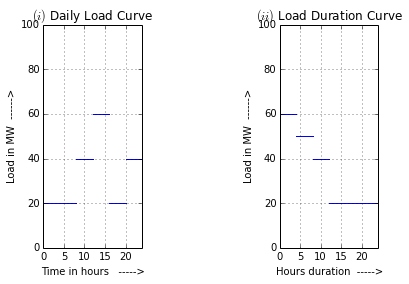### Example 3.16, Page Number: 57¶

In :
from __future__ import division

#Variable declaration:
c1 = 10                  #Capacity of each of 2 generators(MW)
c2 = 5                   #Capacity of 3rd generator(MW)

#Calculation:
C = 2*c1+c2              #Installed capacity(MW)
A = (1/2)*(20+4)         #Avg load, seen from the curve(MW)
PF = A/C                  #plant factor
E = 8760*A               #Units generated per annum(MWh)
PUF = 20/C               #Plant capacity factor

#Results:
print "(i) Installed capacity is ",C,"MW"
print "(ii) Plant factor is ",round(PF*100,1),"%"
print "(iii) Units generated per annum is ",(E/1000),"* 10^3  kWh"
print "(iv) Load factor is ",round(LF*100,1),"%"
print "(V) Utilisation factor is ",round(PUF*100,1),"%"

(i) Installed capacity is  25 MW
(ii) Plant factor is  48.0 %
(iii) Units generated per annum is  105.12 * 10^3  kWh
(iv) Load factor is  60.0 %
(V) Utilisation factor is  80.0 %


### Example 3.17, Page Number: 57¶

In :
#Variable declaration:
n = 0.72                      #efficiency of the load

#Calculation:
Ma = (10*0.746/0.72)* 0.65 + 5 * 0.60       #kW
Mb = (7.5*0.746/0.72)*0.75+4*0.60           #kW
Mc = (15*0.746/0.72)*0.65                    #kW
Md = (5*0.746/0.72)*0.75+2*0.60              #kW

SM = Ma+Mb+Mc+Md                            #Sum of maximum demands(kW)

#The diversity factor between consumers of this type of service is 1·5 from above table.
M1 = SM/1.5                                  #Maximum demand on transformer 1(kW)

#In a similar manner, the other transformer loads are determined to be
#                Total       Simultaneous
#Transformer 2   26 kW        7·43 kW
#Transformer 3   29·13 kW    19·40 kW

#The diversity factor between transformers is 1·3
M2 = 7.43; M3 = 19.40
MF = (M1+M2+M3)/1.3                     #Maximum load on feeder(kW)

#Results:
print "The maximum load on the feeder is ",round(MF,2),"kW"

The maximum load on the feeder is  37.64 kW


### Example 3.18, Page Number: 61¶

In :
from __future__ import division
%matplotlib inline

#Calculation:
E = 10**3*(20*2+40*3+50*5+35*3+70*3+40*2+20*6)       #Units generated per day(kWh)

n1 = linspace(0,8,10);
m1 = linspace(20,20,10);
plot(n1,m1);

hold(True);
n2 = linspace(8,11,10);
m2 = linspace(40,40,10);
plot(n2,m2,'b');

n3 = linspace(11,16,10);
m3 = linspace(50,50,10);
plot(n3,m3,'b');

n4 = linspace(16,19,10);
m4 = linspace(35,35,10);
plot(n4,m4,'b');

n5 = linspace(19,22,10);
m5 = linspace(70,70,10);
plot(n5,m5,'b');

n6 = linspace(22,24,10);
m6 = linspace(40,40,10);
plot(n6,m6,'b');

ylim(0,100);
xlim(0,24);
grid(linewidth=0.5);
xlabel("Time in hours   ----->");

#Results:
print """\nOperational schedule: Referring to the load curve shown
below, the operational schedule will be as under :
(i)  Set No. 1 will run for 24 hours.
(ii) Set No. 2 will run from 8.00 hours to midnight.
(iii)Set No. 3 will run from 11.00 hours to 16 hours and
again from 19 hours to 22 hours."""
print "\nThe Load curve is shown below:"

Populating the interactive namespace from numpy and matplotlib

Operational schedule: Referring to the load curve shown
below, the operational schedule will be as under :
(i)  Set No. 1 will run for 24 hours.
(ii) Set No. 2 will run from 8.00 hours to midnight.
(iii)Set No. 3 will run from 11.00 hours to 16 hours and
again from 19 hours to 22 hours.

The Load curve is shown below:

WARNING: pylab import has clobbered these variables: ['tri', 'axes', 'legend', 'stackplot', 'figure', 'rc_context', 'quiver', 'streamplot', 'rc', 'text', 'colors', 'contour', 'axis', 'colorbar', 'test', 'rcdefaults', 'table']
%pylab --no-import-all prevents importing * from pylab and numpy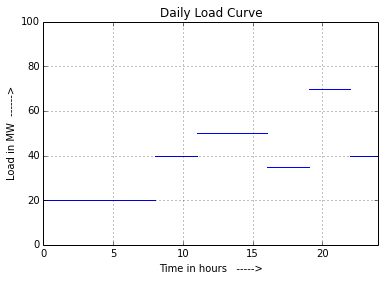### Example 3.19, Page Number: 61¶

In :
#Variable declaration:
P1 = 10
P2 = 5
P3 = 8
P4 = 7

DF = 1.5                   #Diversity factor
LF = 0.60                  #Avg annual load factor

#Calculation:
M = (P1+P2+P3+P4)/DF        #Max demand on the station(MW)
E = (LF*M)*8760             #Annual energy supplied(MWh)
#the installed capacity should be 15% to 20% more than the
#maximum demand to meet the future needs.
#Taking Installed capacity to be 20% more than the maximum demand,
IC = 1.2*M                  #MW

#Results:
print "(i)  The maximum demand on the station is",M,"MW"
print "(ii) Annual energy supplied by the station is (",E/1000,"* 10^6)  kWh"
print "(iii)Installed capacity is",IC,"MW"
print "     Suitable unit sizes are 4, each of 6 MW capacity."

(i)  The maximum demand on the station is 20.0 MW
(ii) Annual energy supplied by the station is ( 105.12 * 10^6)  kWh
(iii)Installed capacity is 24.0 MW
Suitable unit sizes are 4, each of 6 MW capacity.


### Example 3.20, Page Number: 64¶

In :
from __future__ import division

#Variable declaration:
c1 = 20                   #capacity of standby station(MW)
c2 = 18                   #Capacity of base load station(MW)
E1 = 7.35*10**6            #Annual standby output(kWh)
E2 = 101.35*10**6          #Annual base load station output(kWh)
P3 = 12                     #peak load on standby station(MW)
t = 2190                  #hours of use by standby station/year

#Calculation:
LF1 = E1/(t*P3*10**3)          #Annual load factor of standby station
PCF1 = E1/(8760*c1*10**3)      #Plant capacity factor of standby station
PCF2 = LF2                      #Plant capacity factor of base load station

#Results:
print "Annual load factor of standby station is",round(LF1*100,1),"%"
print "Plant capacity factor of standby station is",round(PCF1*100,1),"%"
print "Plant capacity factor of base load station is",round(PCF2*100,1),"%"

Annual load factor of standby station is 28.0 %
Plant capacity factor of standby station is 4.2 %
Plant capacity factor of base load station is 64.3 %


### Example 3.21, Page Number: 64¶

In :
from sympy import *

#Variable declaration:
M = 60000                   #maximum load of hydro plant(kW)
m = 20000                   #minimum load of hydro pland(kW)
P = 50000                    #peak load of plant per day(kW)

#Calculation:
#Let OE = Capacity of steam plant
#EC = Capacity of hydro plant
x,y = symbols('x,y')            #two variables as indicated in given curve
# As steam electric conversion is 60%,
#∴ Area HEFI = 0·6 × Area FGB                            ... (i)
#But Area HEFI = Area CFE − Area CHI
#              = 1/2*(x*y-50000)
#  Now Area FGB = 1/2*(FG*GB) = 1/2*(24-x)*(40000-y)
#  or 0.2*x*y + 12000*x + 7.2*y − 338000 = 0
#Also from similar triangles CEF and CDB, we get,
#     y/40000 = x/24
y = x/24*40000
x1 = round(solve(0.2*x*y + 12000*x + 7.2*y-338000,x))
y1 = x1/24*40000

#Result:
print "Capacity of the hydro plant is",y1,"kW"
print "Capacity of the steam plant is",60000-y1,"kW"

Capacity of the hydro plant is 20000.0 kW
Capacity of the steam plant is 40000.0 kW


### Example 3.22, Page Number: 65¶

In :
from __future__ import division
from sympy import *

#Variable declaration:
#Suppose the maximum demand of reservoir plant is y MW and it
#operates for x hours
x,y = symbols('x y')

#Calculation:
E = 10**3*(1/2*(320+160)*8760)            #Units generated/annum
#As the steam plant, run-of-river plant and hydro plant generate
#units in the ratio of 7 : 4 : 1, so, units generated by
#each plant are:

E1 = E*7/12                    #by steam plant
E2 = E*4/12                    #by run-of-river plant
E3 = E/1/12                    #by reservoir plant

#(i) Maximum demand on run-of-river plant = Area OEBA/OA
M2 = 700.8*10**6/8760           #kW

#Now,
x = 8760*y/160
E33 = 10**3*1/2*(x*y)
#But the units generated by reservoir plant are E3 kWh
y1 = solve(E33-E3,y)
M3 = y1                    #maximum demand on reservoir station(MW)
M1 = 320-80-M2/1000              #Maximum demand on steam station(MW)
LF2 = 100            #load factor of river plant(%), because it acts
#as a base station.

LF1 = E1*100/(M1*1000*8760)         #load factor of of run-of-river plant(%)
LF3 = E3*100/(M3*1000*8760)           #load factor of reservoir plant(%)

#Result:
print "(i)Maximum demand on run-of-river plant is",round(M1),"MW"
print "   Maximum demand on steam station is",round(M2),"MW"
print "   Maximum demand on reservoir station is",round(y1),"MW"
print "\n(ii)Load factor of steam plant plant is",LF1,"%"
print "    Load factor of of run-of-river plant is",LF2,"%"
print "    Load factor of of reservoir plant is",round(LF3),"%"

(i)Maximum demand on run-of-river plant is 160.0 MW
Maximum demand on steam station is 80000.0 MW
Maximum demand on reservoir station is 80.0 MW

(ii)Load factor of steam plant plant is 87.5 %
Load factor of of run-of-river plant is 100 %
Load factor of of reservoir plant is 25.0 %